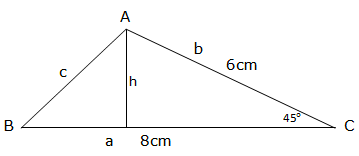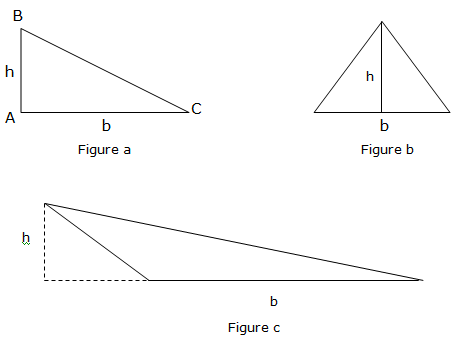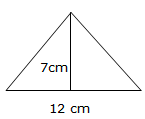Posted on

# AREA AND PERIMETERUNAWEZA JIPATIA NOTES ZETU KWA KUCHANGIA KIASI KIDOGO KABISA:PIGA SIMU:0787237719

Area of any Triangle
The Formula for the Area of any Triangle
Derive the formula for the area of any triangle
Area of triangle is given by½bh, whereby b is the base of the triangle and h is the height of the given triangle. Consider the illustrations below:From the figure above, we see where the base and height are located.
Applying the Formula to find the Area of any Triangle
Apply the formula to find the area of any triangle
Example 1
The base of a triangle is 12cm long. If the corresponding height is 7cm, find the area of the triangle.
Solution
Consider the figure below:The area of a triangle is given by½bh.
Area =½×12cm×7cm
Area = 42cm2
Therefore area of a triangle is 42cm2
Example 2
The lengths of two sides of a triangle are 6cm and 8cm. Find the area of a triangle if the included is
Solution:
Consider the triangle below, name it triangle ABC.The area of a triangle above is given by½b×h
So, Area = ½× 8cm× 6cm × sin 45°
=24cm2× sin45°
= 16.97cm2
Therefore the area of ABC = 16.97cm2

### Dear our readers and users you can also navigate our all study notes in our site though this post please to read our notes by classes click lick button down

JE UNAMILIKI SHULE AU BIASHARA NA UNGEPENDA IWAFIKIE WALIO WENGI?BASI TUNAKUPA FURSA YA KUJITANGAZA NASI KWA BEI NAFUU KABISA BOFYA HAPA KUJUA

But for more post and free books from our site please make sure you subscribe to our site and if you need a copy of our notes as how it is in our site contact us any time we sell them in low cost in form of PDF or WORD.

UNAWEZA JIPATIA NOTES ZETU KWA KUCHANGIA KIASI KIDOGO KABISA:PIGA SIMU:0787237719

SHARE THIS POST WITH FRIEND